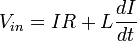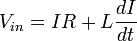# Help With Inductance

## Homework Statement

A inductor that has a resistance of 80 k-Ohms is connected to an ideal battery of 84V. 0.8 milliseconds after the switch is thrown the current in the circuit is .5355 mA. Calculate the Inductance. answer in units of H.

V = L(I/t)?

## The Attempt at a Solution

Well at first I thought there was a voltage drop when the switch was thrown, I calculated that at 42.84V after the switch is thrown. But I have no idea if that's even right.

Any guidance or help will be greatly appreciated.

Try writing the differential equation for a battery, inductor, and resistor all in series, with current flow starting when a switch closes the circuit. Solve the differential equation and see if this does not get you going.

This is the only differential equation I know for an inductor in series with a resistor.I'll keep trying and reading my book though, I've been working on this problem for the last two hours thoughYou have not included the battery. Don't you know Kirchoff's Voltage Law? Apply that for this case. You have part of the necessary expression here, but put it all together as a D.E. for the circuit.

Actually I believe he has his battery voltage right there as Vin.

First you gotta specify your initial conditions more clearly. Was the inductor attached to the battery prior to t=0 or was the battery connected at t=0? Or was the battery disconnected at t=0?

This is important as it will define your initial conditions for the DE.

This is the only differential equation I know for an inductor in series with a resistor.I'll keep trying and reading my book though, I've been working on this problem for the last two hours thoughThats it... Solve the DE and your job is done

Its not right when you don't recognize that Vin is the battery voltage. Until that point, it is wrong. (Battery voltages are usually denoted by those who recognize them as such, as E, rather than Vin.)

Its not right when you don't recognize that Vin is the battery voltage. Until that point, it is wrong. (Battery voltages are usually denoted by those who recognize them as such, as E, rather than Vin.)

Fair enough